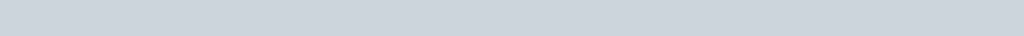# How to load data in Python using Scikit-Learn?

This tutorial will show you how to load data sets in Python using Scikit-Learn (and also Pandas library).

Basically, we will learn how to load data sets using 3 different methods:

1. Using Scikit-Learn’s pre-loaded data sets (By using Scikit-Learn)
2. Importing the data set from the local CSV file (By using Pandas)

## Method 1: Scikit-Learn’s pre-loaded data sets

Scikit-Learn is a handy library used for Machine Learning. In this tutorial, we will learn about the pre-existing libraries present in Scikit-Learn. They are called Toy Datasets. They are primarily used for practice purposes.

#### Here is the list of some famous toy datasets:

1. Boston House Prices
2. Breast Cancer
3. Iris
4. Diabetes
5. Digits

These are some of the few datasets we use in Scikit-learn. These are very famous; hence, we will be loading the dataset ‘Breast Cancer’ from one of these datasets.

We begin with the dataset module as given in the Scikit-learn library.

```from sklearn.datasets import load_breast_cancer

#We will print the keys present in the breast cancer dataset
print(cancer_data.keys())```

Output:After getting the keys, we will focus on getting the description of the dataset. Here, we write the following piece of code.

```#Now we print the description of the dataset
print(cancer_data['DESCR'])```

Output:After getting the output, we will prepare our dataset, after we have gathered it. Here the whole data will be put into the datagram and the column names will come from the feature names, which you can check out from the keys.

```import pandas as pd

df = pd.DataFrame(cancer_data['data'], columns = cancer_data['feature_names'])We will do some linear regression operations on it:

```#We will do a basic logistic regression model

from sklearn.linear_model import LogisticRegression```

We will set up the data for fitting on the best line. We will create another column called target which inserts the target values of the key of the dataset (check the keys again to understand).

```x = df[cancer_data.feature_names].values
df['target'] = cancer_data['target']
y = df['target'].values```

We will fit the data on the best-fit line.

```model = LogisticRegression(solver = 'liblinear')
model.fit(x,y)```

Here, since it exceeds the number of iterations, we have now set the solver argument to ‘liblinear’.\

`model.score(x,y)`

After using the score function, we get the output:

0.9595782073813708

## Method 2: Importing Dataset from local CSV file

For importing the dataset from a local CSV file, we will have to first create an excel sheet where we will put our data.

Here is a sample Excel Sheet:Hence, we will first copy its location and then we will put it into our data frame. Here, we will use the Pandas library for loading the CSV file. We write the following code:

```import pandas as pd

df```

Here, we used ‘r’ before loading the data set because it tells the compiler that the data set is from the local device.

Hence we get the following output:We can even pick up data sets from a given URL. For this, we will again use the Pandas library to load the dataset.

We will use the Pima Indians diabetes classification dataset.

We will write the following piece of code using the read_csv function from the Pandas library:

```url = "https://raw.githubusercontent.com/jbrownlee/Datasets/master/pima-indians-diabetes.data.csv"